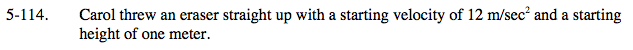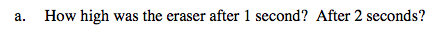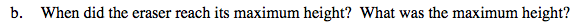### Home > CALC > Chapter 5 > Lesson 5.3.1 > Problem5-114

5-114.Notice that the units are in meters, not feet. Which generic falling object (in earth) equation should we use?

$s(t)=-\frac{32}{2}t^{2}+v_{0}t+s_{0} \ \ \ \ \ \ \text{ or } \ \ \ \ \ \ s(t)=-\frac{9.8}{2}t^{2}+v_{0}t+s_{0}$

Set up the generic falling object (on Earth) equation, plugging in values for initial velocity, v0; and initial position, s0.

Evaluate at t = 1 and t = 2.Optimize the position.

Be sure to answer both questions.